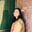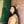Trusted answers to developer questions
Trusted Answers to Developer Questions

Related Tags

numpy
python

# What is numpy.maximum() in Python?Umme Ammara

Grokking Modern System Design Interview for Engineers & Managers

Ace your System Design Interview and take your career to the next level. Learn to handle the design of applications like Netflix, Quora, Facebook, Uber, and many more in a 45-min interview. Learn the RESHADED framework for architecting web-scale applications by determining requirements, constraints, and assumptions before diving into a step-by-step design process.

Python’s numpy.maximum() computes the element-wise maximum of an array. It compares two arrays and returns a new array containing the maximum values. The illustration below shows this functionality:

## Syntax

numpy.maximum() is declared as follows:

numpy.maximum(x1, x2, /, out=None, *, where=True, casting='same_kind', order='K', dtype=None, subok=True[, signature, extobj]) = <ufunc 'maximum'>


In the syntax given above, x1 and x2 are non-optional parameters and the rest are optional parameters.

A universal function (ufunc) is a function that operates on ndarrays in an element-by-element fashion. The maximum() method is a universal function.

## Parameters

The numpy.maximum() method takes the following compulsory parameters:

• x1 and x2 [array-like] - arrays holding the values that need to be compared. If the shapethe shape of an array is the number of elements in each dimension of x1 and x2 is different, they must be broadcastable to a common shape for representing the output.

The numpy.maximum() method takes the following optional parameters:

 Parameter Description out represents the location into which the output of the method is stored where True value indicates that a universal function should be calculated at this position casting controls the type of datacasting that should occur. The same_kind option indicates that safe casting or casting within the same kind should take place. order controls the memory layout order of the output function. The option K means reading the elements in the order they occur in memory dtype represents the desired data type of the array subok decides if subclasses should be made or not. If True, subclasses will be passed through.

## Return value

numpy.maximum() returns the maximum of x1 and x2 element-wise. The return type is ndarray or scaler depending on the input.

If one of the elements being compared is NaN (Not a Number), then NaN is returned.

If both elements being compared are NaN (Not a Number),then NaN is returned.

## Examples

The examples below show different ways in which numpy.maximum() is used in Python.

The following code example outputs the maximum of two numbers a and b:

import numpy as npa = 10b = 20print (np.maximum(a,b))

The example below outputs the result of comparing the arrays arr1 and arr2:

import numpy as nparr1 = np.array([1,3,4,np.nan])arr2 = np.array([np.nan,6,2,np.nan])print(np.maximum(arr1,arr2))

The example below shows the result of comparing the arrays arr3 and arr4:

import numpy as nparr3 = np.array([[1,2,3], [4,5,6]])arr4 = np.array([[3,2,1], [6,5,4]])print(np.maximum(arr3,arr4))

RELATED TAGS

numpy
python

CONTRIBUTORUmme Ammara